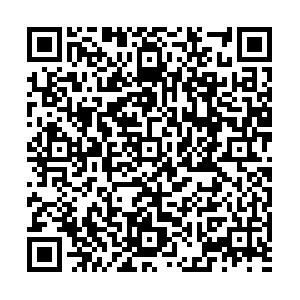# J/ψ-J/ψ scattering cross sections of quadratic and Cornell potentials• We study the scattering of $J/\psi$- $J/\psi$mesons using quadratic and Cornell potentials in our tetraquark (${{c\bar cc\bar c}}$) system. The system’s wavefunction in the restricted gluonic basis, which is written by utilizing the adiabatic approximation and Hamiltonian, is used via a quark potential model. The resonating group technique is used to obtain the integral equations, which are solved to obtain the unknown inter-cluster dependence of the total wavefunction of our tetraquark system. T-Matrix elements are calculated from the solutions, and eventually, the scattering cross sections are obtained using the two potentials. We compare these cross sections and find that the magnitudes of scattering cross sections of quadratic potential are higher than the Cornell potential.
••Get Citation
M. Imran Jamil, S.M. Sohail Gilani, Ahmad Wasif, Abdul Sattar Khan and Ahmad Awan. J/Ψ-J/Ψ scattering cross sections of Quadratic and Cornell Potentials[J]. Chinese Physics C. doi: 10.1088/1674-1137/abcc5b
M. Imran Jamil, S.M. Sohail Gilani, Ahmad Wasif, Abdul Sattar Khan and Ahmad Awan. J/Ψ-J/Ψ scattering cross sections of Quadratic and Cornell Potentials[J]. Chinese Physics C.Milestone
Revised: 2020-09-22
Article Metric

Article Views(215)
Cited by(0)
Policy on re-use
To reuse of Open Access content published by CPC, for content published under the terms of the Creative Commons Attribution 3.0 license (“CC CY”), the users don’t need to request permission to copy, distribute and display the final published version of the article and to create derivative works, subject to appropriate attribution.
###### 通讯作者: 陈斌, bchen63@163.com
• 1.

沈阳化工大学材料科学与工程学院 沈阳 110142

Title:
Email:

## J/ψ-J/ψ scattering cross sections of quadratic and Cornell potentials

• 1. Department of Physics, School of Science, University of Management and Technology, Lahore (54770), Pakistan
• 2. Department of Physics,University of Okara, Okara (56300), Pakistan
• 3. Department of Physics, Forman Christian College (A Chartered University), Lahore (54600), Pakistan
• 4. Department of Physics, Government College University, Lahore (54000), Pakistan

Abstract: We study the scattering of $J/\psi$- $J/\psi$mesons using quadratic and Cornell potentials in our tetraquark (${{c\bar cc\bar c}}$) system. The system’s wavefunction in the restricted gluonic basis, which is written by utilizing the adiabatic approximation and Hamiltonian, is used via a quark potential model. The resonating group technique is used to obtain the integral equations, which are solved to obtain the unknown inter-cluster dependence of the total wavefunction of our tetraquark system. T-Matrix elements are calculated from the solutions, and eventually, the scattering cross sections are obtained using the two potentials. We compare these cross sections and find that the magnitudes of scattering cross sections of quadratic potential are higher than the Cornell potential.

### HTML关注分享DownLoad:  Full-Size Img  PowerPoint